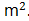### Sample Problem

The average area of a rectangle and a triangle is 40. The triangle has a base of 8 m and a height of 9 m. If the rectangle has a width of 4 m, what is its perimeter?

m

#### Solution

The total area of the two shapes is 40 × 2 = 80.

The area of the triangle is 1/2 × base × height = 1/2 × 8 × 9 = 36The area of the rectangle is total area – area of the triangle 80 – 36 = 44The length of the rectangle is area ÷ width = 44 ÷ 4 = 11 m.

Therefore, the perimeter of the rectangle is 11 × 2 + 4 × 2 = 30 m.